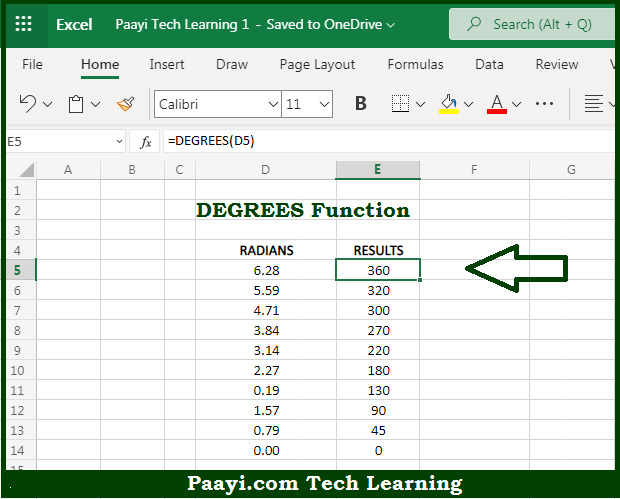# Learn How to Use Microsoft Excel DEGREES Function

Written by | 0 Comments | 555 Views

In this article, you will learn how to use the Microsoft Excel DEGREES function and its prime function in Microsoft Excel. You will also get to know the Microsoft Excel DEGREES function return value and syntax with the help of some examples.

Microsoft Excel DEGREES Function

The main purpose of the Microsoft Excel DEGREES function is to convert the radians into degrees. That implies, with the help of the DEGREES function you can able to convert the angles provided in radians to degrees. So, with the help of the DEGREES function, you can able to convert the radians into degrees.

Return Value of DEGREES Function

The return value will be the degrees.

Syntax of DEGREES Function

=DEGREES(angle)

Where the arguments:

• angle: This is the angle provided in radians, which you want to convert in degrees.

How to Use Microsoft Excel DEGREES Function?So we know that Microsoft Excel DEGREES function you can able to convert the radians into degrees. That implies, with the help of the DEGREES function you can able to convert the angles provided in radians to degrees. So, with the help of the DEGREES function, you can able to convert the radians into degrees.